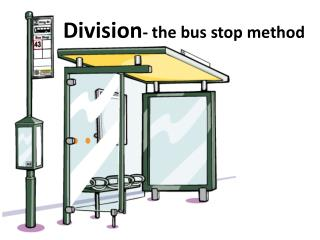Download PresentationDivision - the bus stop method

# Division - the bus stop method - PowerPoint PPT Presentation

Division - the bus stop method. Warm up questions. 10 5 7 6 4 5 4 7 6 4 3 7 7 3 3 8 9 12 9 5 12 9 4 5 12 8 12 12 11 11. 30÷3= 25÷5= 42÷6= 18÷3= 24÷6= 15÷3= 28÷7= 49÷7= 36÷6= 16÷4= 24÷8= 21÷3= 14÷2= 12÷4= 36÷12= 56÷7= 81÷9= 72÷6= 90÷10= 40÷8=I am the owner, or an agent authorized to act on behalf of the owner, of the copyrighted work described.
Download Presentation## Division - the bus stop method

An Image/Link below is provided (as is) to download presentation

Download Policy: Content on the Website is provided to you AS IS for your information and personal use and may not be sold / licensed / shared on other websites without getting consent from its author.While downloading, if for some reason you are not able to download a presentation, the publisher may have deleted the file from their server.

- - - - - - - - - - - - - - - - - - - - - - - - - - E N D - - - - - - - - - - - - - - - - - - - - - - - - - -
Presentation Transcript
1. Division- the bus stop method

2. Warm up questions 10 5 7 6 4 5 4 7 6 4 3 7 7 3 3 8 9 12 9 5 12 9 4 5 12 8 12 12 11 11 • 30÷3= • 25÷5= • 42÷6= • 18÷3= • 24÷6= • 15÷3= • 28÷7= • 49÷7= • 36÷6= • 16÷4= • 24÷8= • 21÷3= • 14÷2= • 12÷4= • 36÷12= • 56÷7= • 81÷9= • 72÷6= • 90÷10= • 40÷8= • 48÷4= • 63÷7= • 32÷8= • 20÷4= • 60÷5= • 64÷8= • 108÷9= • 144÷12= • 121÷11= • 110÷10=

3. =53 159 ÷ 3 5 3 0 1 3 1 5 9 1÷3=0 remainder 1 15÷3=5 9÷3=3

4. =57 285 ÷ 5 5 7 0 3 2 5 2 8 5 2÷5=0 r 2 35÷5=7 28÷5=5 r 3

5. =24 288 ÷ 12 2 4 0 4 2 12 2 8 8 48÷12=4 2÷12=0 r 2 28÷12=2 r 4

6. =1.36 5.44 ÷ 4 3 6 1 . 2 1 4 5 4 4 . 14÷4=3 r 2 5÷4=1 r 1 24÷4=6

7. =5.4 27 ÷ 5 5 4 0 . 2 2 5 2 7 0 . 20÷5=4 2÷5=0 r 2 27÷5=2 r 2

8. =4.25 34 ÷ 8 4 2 0 5 . 4 2 3 0 4 8 3 0 . 3÷8=0 r 3 34÷8=4 r 2 20÷8=2 r 4 40÷8=5

9. Practise Questions B- I want practise working with decimals 98÷8 65÷4 14÷5 194÷8 145÷4 119.9÷7 138.6÷9 108.5÷5 356.4÷11 195.9÷3 C- I want a challenge 432÷18 465÷15 529÷23 966÷23 864÷27 575÷25 924÷28 544÷16 665÷19 756÷18 A- I want to practise the “basics” • 284÷4 • 201 ÷ 3 • 612÷12 • 504÷7 • 294÷7 • 335÷5 • 329÷7 • 957÷11 • 522÷6 • 712÷8 =71 =67 =51 =72 =82 =67 =47 =87 =87 =89 =24 =31 =23 =42 =32 =23 =33 =34 =35 =42 =12.25 =16.25 =2.8 =24.25 =36.25 =16.7 =15.4 =21.7 =32.4 =65.3